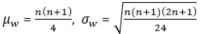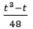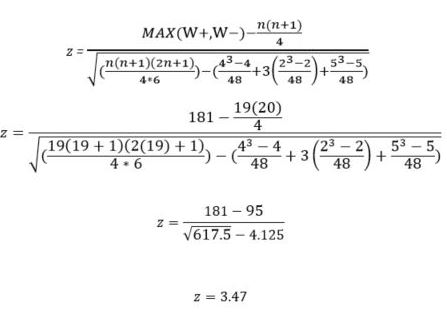The Wilcoxon signed rank test is the non-parametric of the dependent samples t-test.  Because the dependent samples t-test analyzes if the average difference of two repeated measures is zero, it requires metric (interval or ratio) and normally distributed data; the Wilcoxon sign test uses ranked or ordinal data; thus, it is a common alternative to the dependent samples t-test when its assumptions are not met.The Wilcoxon signed rank test relies on the W-statistic.  For large samples with n>10 paired observations the W-statistic approximates a normal distribution.  The W statistic is a non-parametric test, thus it does not need multivariate normality in the data.

A research team wants to test whether a new teaching method increases the literacy of children.  Therefore, the researchers take measure the literacy of 20 children before and after the teaching method has been applied.  The literacy is measured on a scale from 0 to 10, with 10 indicating high literacy.  The initial baseline shows an average literacy score of 5.9 and after the method has been used the average increases to 7.6.

A dependent samples t-test cannot be used, as the distribution does not approximate a normal distribution.  Also both measurements are not independent from each other; therefore, the Mann-Whitney U-test cannot be used.

The first step of the Wilcoxon sign test is to calculate the differences of the repeated measurements and to calculate the absolute differences.The next step of the Wilcoxon sign test is to sign each rank.  If the original difference < 0 then the rank is multiplied by -1; if the difference is positive the rank stays positive.For the Wilcoxon signed rank test we can ignore cases where the difference is zero.  For all other cases we assign their relative rank. In case of tied ranks the average rank is calculated.  That is if rank 10 and 11 have the same observed differences both are assigned rank 10.5.

The next step of the Wilcoxon sign test is to sign each rank.  If the original difference < 0 then the rank is multiplied by -1; if the difference is positive the rank stays positive.The next step is to calculate the W+ and W-.

W+ = 4.5 + 4.5 = 9

W- = 1+ 2 + 4.5 + 4.5 + 7.5 + 7.5 + 9 + 10.5 + 10.5 + 12.5 + 12.5 + 14 + 17+ 17 + 17 + 17 + 17 = 181

We can double check this knowingFor large samples with n>10 the W-statistics approximates normal distribution, withFurther, we must make a compensation for ties, 4, 2, 2, 2, and 5, so we must reduce the variance byThe shortcut to the hypothesis testing of the Wilcoxon signed rank-test is knowing the critical z-value for a 95% confidence interval (or a 5% level of significance) which is z = 1.96 for a two-tailed test and directionality.  Whenever a test is based the normal distribution the sample z value needs to be 1.96 or higher to reject the null hypothesis.